Bounty: Uniswap shiny NFTs¶

Is there a way to figure out who got Shiny NFTs? What is the concentration of shiny NFTs in the hands of the 20 largest Liquidity Providers by TVL on Uniswap?

Initialising notebook

In :
from matplotlib import pyplot as plt
#import rare
import pandas as pd
import numpy as np
import requests
import math
from web3 import Web3
from IPython.display import Image
import warnings
warnings.filterwarnings("ignore")

Importing position data from Flipside

In :
url = 'https://api.flipsidecrypto.com/api/v2/queries/4c9dcef8-7ca3-4192-8288-6e4354a96300/data/latest'
response = requests.get(url)
df = pd.DataFrame(response.json())

url = 'https://api.flipsidecrypto.com/api/v2/queries/97acbb69-8689-4507-8306-f6a36375cd30/data/latest'
response = requests.get(url)
df2 = pd.DataFrame(response.json())

df = pd.concat([df, df2]).drop_duplicates()

LIQUIDITY_PROVIDER  NF_TOKEN_ID  \

0  0x1d42064fc4beb5f8aaf85f4617ae8b3b5b8bd801
1  0x6c6bc977e13df9b0de53b251522280bb72383700

Defining the reverse-engineered rare NFTs function from Uniswap source code

The function determines whether a particular NFT is rare based on the formula in the image below, we can reverse-engineer this to find which NFTs are rare.

Interestingly, we can see the rarity of shiny NFTs grows as more are minted, with a 'difficulty bomb' every 2^(2^i) NFTs minted (where i is a positive integer)

In :
display(Image(filename='israre.png'))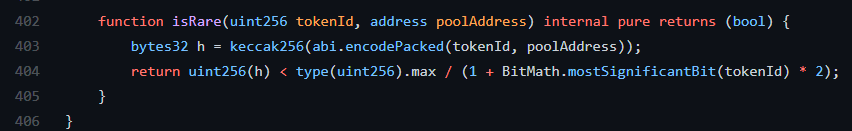Using the Python web3 library we can emulate the function as per below

In :

y = math.log2(x)

temp = 0
for pow in range(1,256):
if y >= 2**pow:
temp += pow

return x <= Web3.toInt(2**256-1)/(1 + temp * 2)

We can now iterate over all the positions to determine which belong to the elusive shiny group

In :
df['rare'] = df.apply(lambda x : rareTest(x['NF_TOKEN_ID'], x['POOL_ADDRESS']), axis = 1)

NF_TOKEN_ID   rare
0            1   True
1            2   True
2            3   True
3            4  False
4            5   True

The first 5 NFTs minted are shiny, with the exception of number 4!

To validate this we can view these NFTs in the Uniswap front-end and lo-and-behold they all have the symbol of rarity *

In :
display(Image(filename='validation.png'))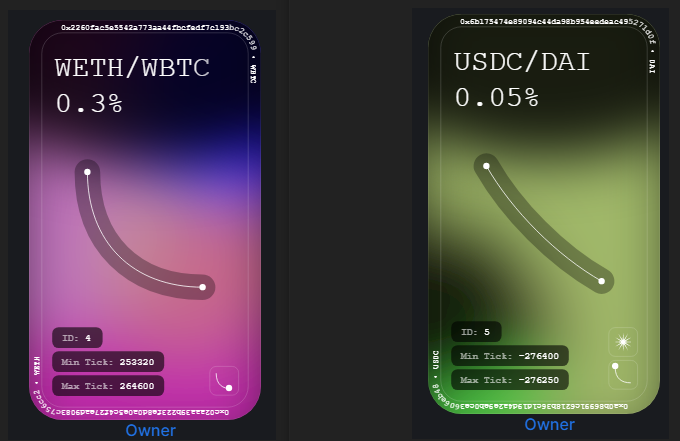Now that we've identified them, how many are there?

In :
string = f'''
There are {df['rare'].sum()} Shiny NFTs
Out of a total of {df['rare'].count()}
Representing a current chance of a shiny NFT at {round(df['rare'].sum()/df['rare'].count()*100,2)}%
'''
print(string)

fig, ax = plt.subplots()
ax.pie([df['NF_TOKEN_ID'].count()-df['rare'].sum(),df['rare'].sum()], labels = ['Normal', 'Shiny'],autopct='%1.1f%%')
plt.show()
There are 7670 Shiny NFTs
Out of a total of 118045
Representing a current chance of a shiny NFT at 6.5%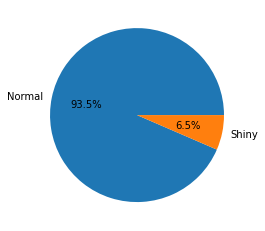Now we can identify how many are owned by the top 20 LPs

I have identified the top 20 LPs by TVL in the top 10 pools using the query below.

In :
url = 'https://api.flipsidecrypto.com/api/v2/queries/5bd38439-1938-4a0a-a781-5e51f8da1181/data/latest'
response = requests.get(url)
whale_df = pd.DataFrame(response.json())
In :
count_df = df[df['LIQUIDITY_PROVIDER'].isin(whale_df['LIQUIDITY_PROVIDER'])].groupby('LIQUIDITY_PROVIDER').agg({'rare':'sum','NF_TOKEN_ID':'count'})
print(count_df)
rare  NF_TOKEN_ID
LIQUIDITY_PROVIDER
0x119454fcd0c84b3e44cec59a718848c70987935f     0            3
0x1578ed833d986c1188d1a998aa5fecd418bef5da     1            2
0x2bb718a3986c36c6e02d8d15cda4370820d08169     0            7
0x342bf4e93930b576b24df05b44dd845305d87e0d     2            6
0x47441bd9fb3441370cb5b6c4684a0104353aec66     0           10
0x66721b617dfdbca40dbc1a92fb0907abb764235a     0            1
0x70fcbd3f1abbc076259b2f200171b067d7ca75b0     0            7
0x741aa7cfb2c7bf2a1e7d4da2e3df6a56ca4131f3     4           83
0x888e1b67bfb11d6a68c43a6af939b89d8defb2bb     0            2
0x8dbe8722557b97a956bac439858d107108cf7b8a     3           18
0x94b1fc7695b9d596e5b65b2b4f3d05d0069bc012     1           19
0x9b0297ce4ebcf554a2000ebd60987298a9bc314b     1           29
0xa0f75491720835b36edc92d06ddc468d201e9b73     0            7
0xd5eeb79bf7325e92bb775c4c642fb20d050f462e     0            3
0xe059e9d45850e5524c075b28b22c5939cc21c94b     0           22
0xe8e8f41ed29e46f34e206d7d2a7d6f735a3ff2cb     0            3
0xf39d30fa570db7940e5b3a3e42694665a1449e4b     0            3
In :
fig2, ax2 = plt.subplots()

ax2.bar(x = count_df.index, height = count_df['NF_TOKEN_ID'] - count_df['rare'], label = 'Normal NFTs')
ax2.bar(count_df.index, count_df['rare'], bottom = count_df['NF_TOKEN_ID'] - count_df['rare'], label = 'Rare NFTs')
plt.xticks(rotation = 90)
plt.legend()
plt.show()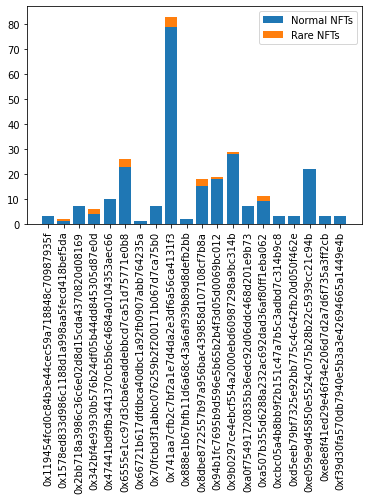In :
string = f'''
In total, the top 20 LPs own {count_df['rare'].sum()} shiny NFTs.
This represents {round(count_df['rare'].sum()/df['rare'].sum()*100,2)}% of all shiny NFTs.
While they own a total of {round(count_df['NF_TOKEN_ID'].sum()/df['NF_TOKEN_ID'].count()*100,2)}% of all NFTs minted.
'''
print(string)
In total, the top 20 LPs own 17 shiny NFTs.
This represents 0.22% of all shiny NFTs.
While they own a total of 0.22% of all NFTs minted

Bonus analysis:¶

How has the rarity of these tokens changed over time?¶

As we saw earlier, 4 of the first 5 tokens were rare! What are the odds? Well as we can see below, a lot higher than they are now.

In :
fig0, ax0 = plt.subplots()
x = [2**0, 2**1, 2**2, 2**4, 2**8, 2**16, 2**32]
p = np.array([1,2,4,8,16,32,64,128,256])
y = []
z = []
for i in x:
temp = sum(p[math.log2(i) >= p])
z.append(temp)
y.append(1/(temp*2+1))

ax0.step(x,y)
ax0.set_xscale('log')
vals = ax0.get_yticks()
ax0.set_yticklabels(['{:,.2%}'.format(x) for x in vals])
ax0.set_xlabel('Token ID')
ax0.set_ylabel('Probability')
ax0.set_title('The probability of receiving a rare token at a given Token ID')
plt.show()
string = f'''
Assuming the encrypted values are uniformly distributed, the current probability of receiving a rare token is {round(y*100,2)}%
The next decrease in probability will occur at token {2**32}, a long way away.
'''
print(string)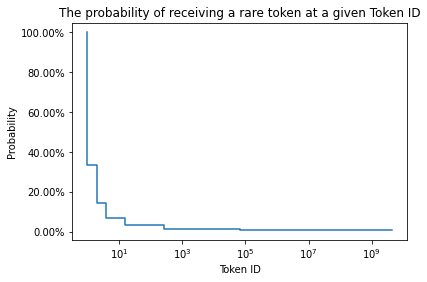Assuming the encrypted values are uniformly distributed, the current probability of receiving a rare token is 0.79%
The next decrease in probability will occur at token 4294967296, a long way away.

Which future tokens are most likely to be the next Shiny?¶

Let's look at the next 100 token IDs

In :
url = 'https://api.flipsidecrypto.com/api/v2/queries/7a690f3e-954c-4025-a5e6-c104b062c969/data/latest'
response = requests.get(url)
position_df = pd.DataFrame(response.json())

position_df['WEIGHT'] = position_df['COUNT']/position_df['COUNT'].sum()
In :
temp = []
for i in range(df['NF_TOKEN_ID'].max(), df['NF_TOKEN_ID'].max()+100):
position_df['temp'] = position_df.apply(lambda x : rareTest(i, x['POOL_ADDRESS']), axis = 1)*position_df['WEIGHT']
temp.append(position_df['temp'].sum())
In :
temp = np.array(temp)
fig, ax = plt.subplots()
ax.plot(range(df['NF_TOKEN_ID'].max(), df['NF_TOKEN_ID'].max()+100),temp)
vals = ax.get_yticks()
ax.set_yticklabels(['{:,.1%}'.format(x) for x in vals])
ax.set_xlabel('Token ID')
ax.set_ylabel('Probability of token being rare')
plt.show()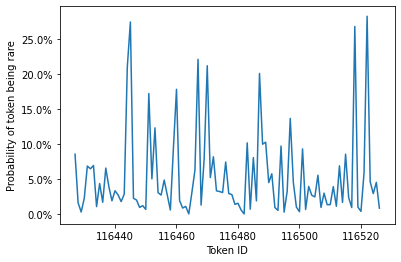As we can see there are spikes in the chart, where a token is more likely to be rare. This appears to peak around 25%. Specific token and address combos make it highly likely for specific tokens to be rare. How curious.

Finally, who is the luckiest of them all?¶

0x335c0552eb130f3dfbe6efcb4d2895aed1e9938b is with 50 shiny NFTs!

In :
x = df[['rare', 'LIQUIDITY_PROVIDER']].groupby(df['LIQUIDITY_PROVIDER']).sum()
print(x.nlargest(1,'rare'))
rare
LIQUIDITY_PROVIDER
0x335c0552eb130f3dfbe6efcb4d2895aed1e9938b    50